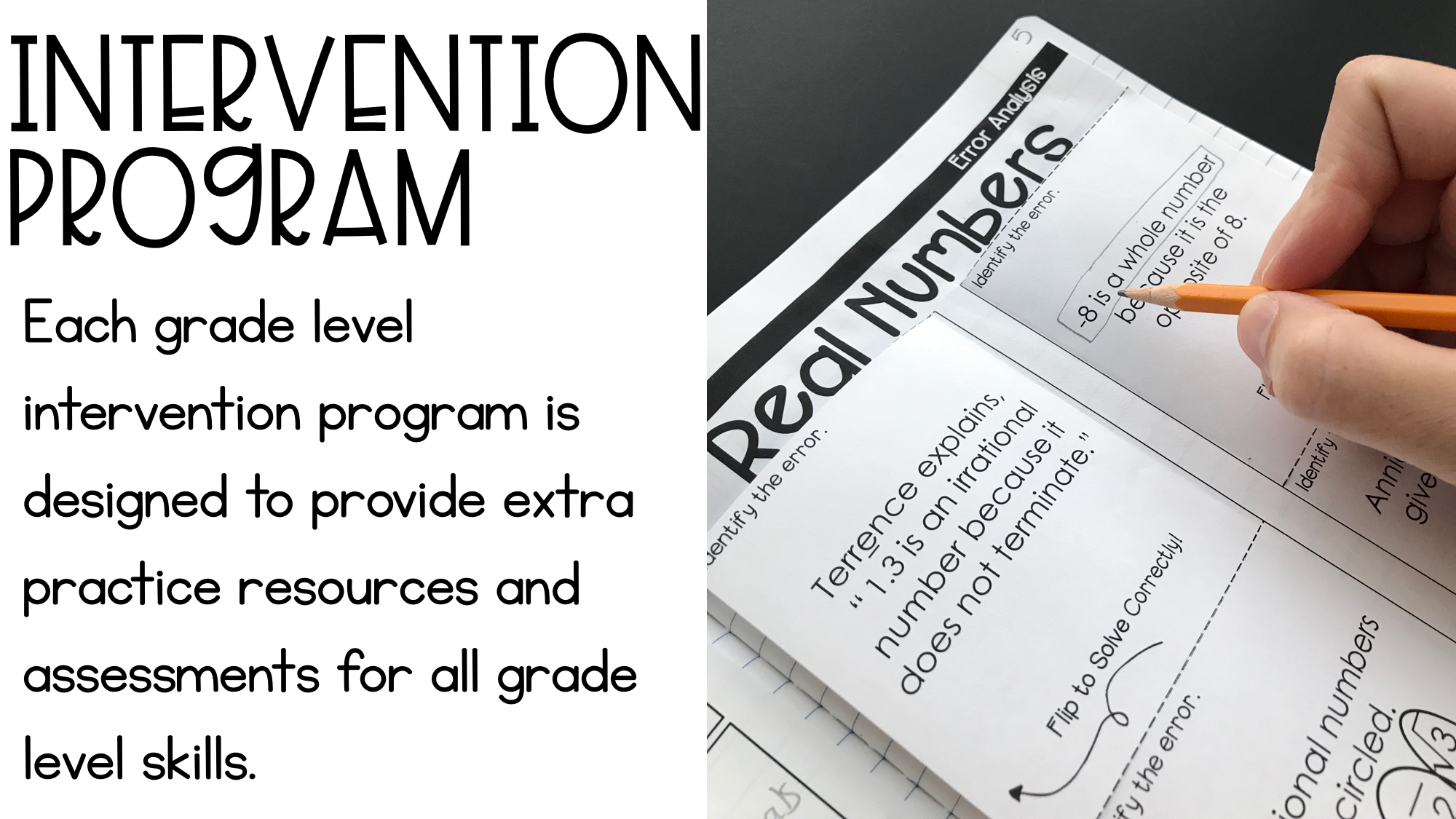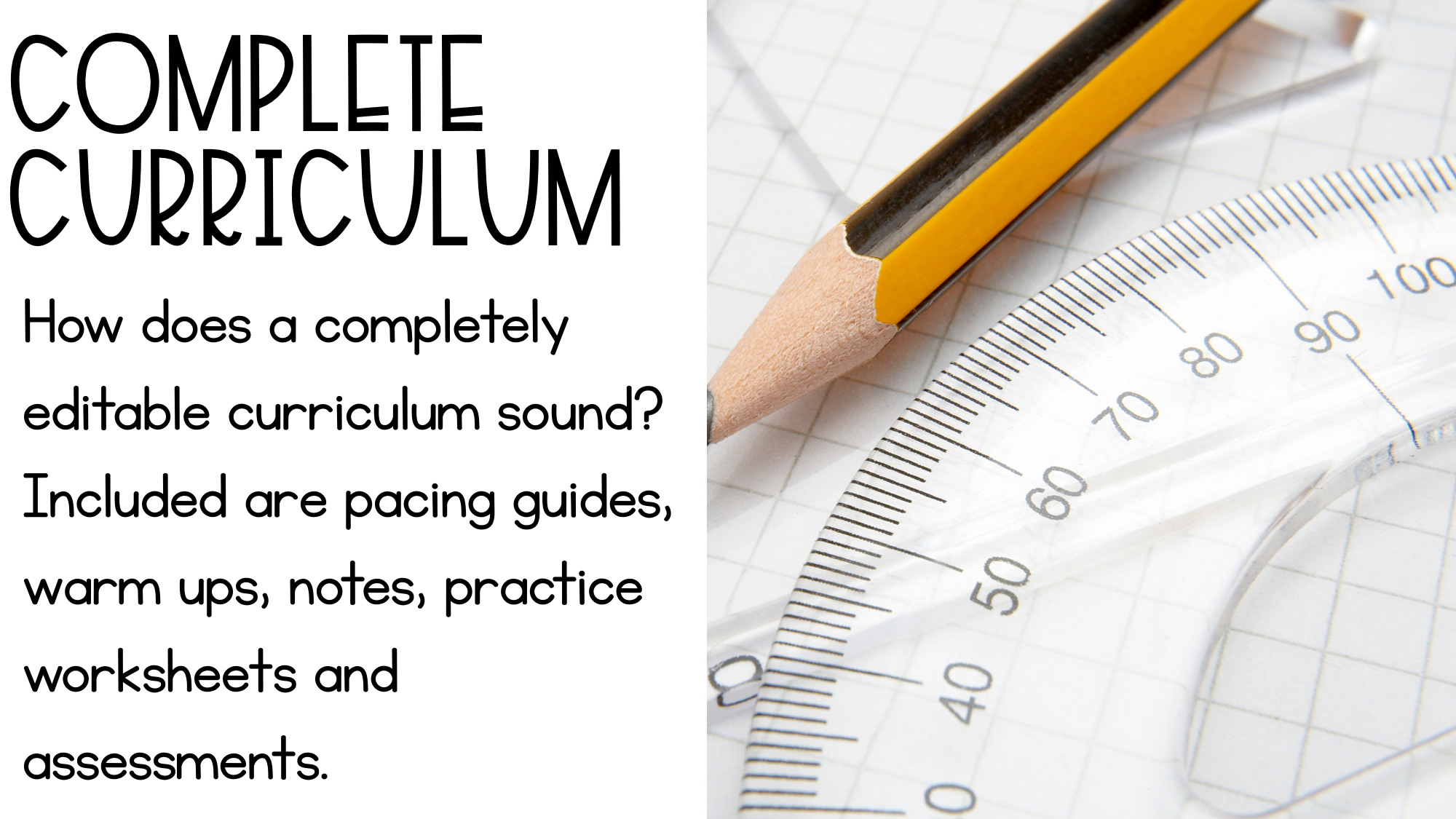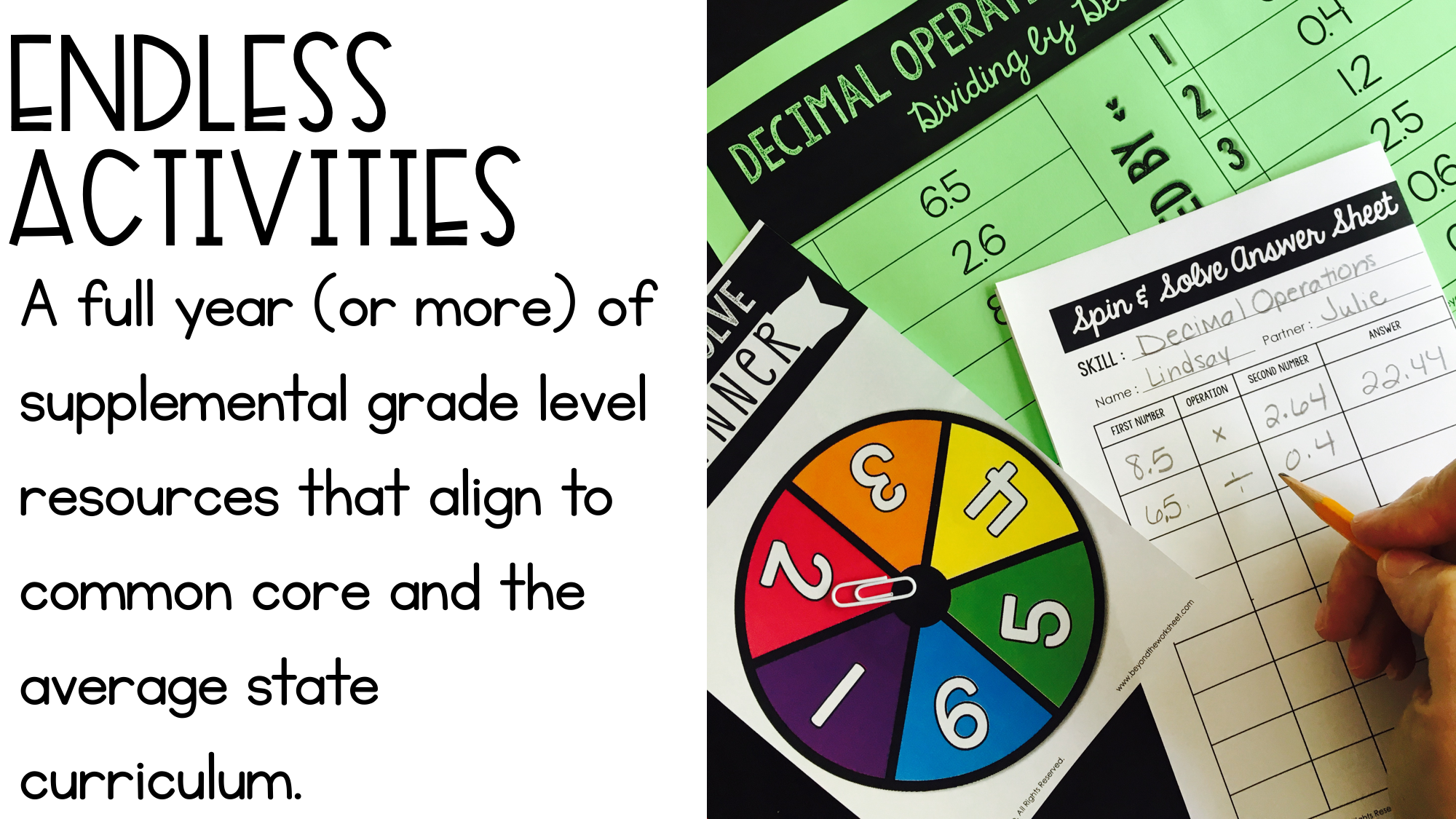# What exactly is the membership?Take back what matters most - your time. For less than the cost of a meal out each month you can have a complete year of curriculum and activities at your fingertips.# Course curriculum

• 1

### START HERE!

• Membership TOUR!

• Rotating Resources Calendar

• 2

### Monthly Bonuses

• Yearly Planner

• Editable Planner Directions

• Editable Yearly Planner

• 3

### Assessments

• 8th Grade Math Benchmark Exams

• 8th Grade Math Final Exam

• 8th Grade Math Final Exam - EDITABLE Version

• 8th Grade Math Mini Assessments (with CCSS)

• 8th Grade Math Mini Assessments (No CCSS)

• 4

### Beginning of the Year Resources

• 8th Grade Math Prep Packet

• 8th Grade Math Prep Packet - HOW TO EDIT

• 8th Grade Math Prep Packet - Editable Version

• Back to School Puzzle Stations

• Back to School Puzzle Stations - Gender Neutral

• Back to School Puzzle Stations - EDITABLE CARDS

• Behavior Data Lesson and Communication Teacher Resources

• Behavior Data Lesson and Communication Teacher Resources EDITABLE FILE

• Editable Syllabus - How to Edit

• Editable Syllabus Editable Pages

• 5

### Escapes (Seasonal Escapes will Rotate)

• Math Escapes Completion Signs

• Solving Higher Level Equations Math Escape

• Solving Higher Level Equations Math Escape - Answer Keys

• Solving Higher Level Equations Math Escape - Challenge Photos

• Solving Equations Escape Higher Level - Editable Files

• Valentine Escape - EDITING and PRINTING Directions

• Valentine Escape - General Escape Directions

• Valentine Escape - Sample Challenge Photos

• Valentine Escape - Completion Signs

• Valentine Escape - Editable Files

• 6

### Full Year Resources

• 8th Grade Math Homework Notebook - Printable Student Copy

• 8th Grade Math Homework Notebook - Printable Teacher Edition

• 8th Grade Homework Notebook - EDITING INFORMATION

• 8th Grade Math Homework Notebook - Editable Student Copy

• 8th Grade I Can Math Punch Cards (CCSS Labeled)

• 8th Grade I Can Math Punch Cards (CCSS Unlabeled)

• 8th Grade I Can Math Punch Cards (CCSS Labeled) - TEMPLATE

• 8th Grade I Can Math Punch Cards EDITABLE FILE (CCSS Labeled)

• 8th Grade I Can Math Punch Cards EDITABLE FILE

• 8th Grade I Can Math Punch Cards - TEMPLATE

• 8th Grade I Can Math Punch Cards (TEKS Labeled)

• 8th Grade I Can Math Punch Cards (TEKS Unlabeled)

• 8th Grade I Can Math Punch Cards - HOW TO EDIT

• 8th Grade I Can Math Punch Cards - TEKS editable files

• 8th Grade Math Bullet Journal

• 8th Grade Math Bullet Journal - Templates

• 8th Grade Math Bullet Notes SAMPLE PAGES

• 8th Grade Math Chats PowerPoint

• 8th Grade Math Chats - HOW TO EDIT

• 8th Grade Math Chats - EDITABLE FILE

• 8th Grade Math Chats Teacher Notes

• 8th Grade Math Quick Reference Sheets

• 8th Grade Quick Reference Sheets - HOW TO EDIT

• 8th Grade Math Quick Reference Sheets - EDITABLE VERSION

• 8th Grade Vocabulary Cards EDITABLE FILE

• 8th Grade Vocabulary Cards TEMPLATE

• 8th Grade Math Common Core Standards Checklists

• Logic Puzzle Bundle

• Middle School Fold and Flip Notes

• Middle School Math Brief Constructed Response Practice

• Middle School Math Writing Prompts

• 7

### Holiday Specific Resources (Will Rotate)

• Editable Heart Themed Puzzle - Valentine's Day

• Editable Winter Coloring Pages

• Pi Day Cylinders Activity

• Summer Logic Puzzles

• Valentines Day Algebra Activity and Coloring Worksheet

• 8

### Intervention

• 8th Grade Math Intervention Binder

• 8th Grade Math Intervention Binder - INB Version

• 8th Grade Math Intervention Binder - Teacher Notes

• 8th Grade Math Intervention Binder - INB Pages & Dividers

• 8th Grade Math Intervention Binder - HOW TO EDIT

• 8th Grade Math Intervention Binder - EDITABLE FILES

• Intervention Plan Sheet

• Intervention Plan Sheet - Editable Version

• Solving Equations Intervention Unit

• Solving Equations Intervention Unit Answer Keys

• 9

### Uncategorized

• Compound Interest Mini Lesson

• Compound Interest Mini Lesson - Answer Keys

• Editable Interactive Notebook Templates - About and Fonts

• Editable Interactive Notebook Templates - Credits

• Editable Interactive Notebook Templates - Cover Pages

• Editable Interactive Notebook Templates - Foldable Note Templates

• Editable Interactive Notebook Templates - Planning Pages

• Editable Interactive Notebook Templates - Side Tab Dividers

• Editable Interactive Notebook Templates - Top Tab Dividers

• Editable Interactive Notebook Templates - TOU

• Editable Interactive Notebook Templates - Editable Files

• Financial Literacy Stations

• Integers Square Roots Pythagorean Theorem Review Game

• Integers Square Roots Pythagorean Theorem Review Game - PowerPoint File

• Math Mistakes in the Real World

• Math Mistakes in the Real World - PowerPoint with Answers

• 10

### Unit 1 Real Numbers and Exponents

• 8th Grade Math Curriculum - Unit 1

• 8th Grade Math Curriculum - Unit 1 Keys

• 8th Grade Math Curriculum - Unit 1 - Student Booklet

• HOW TO EDIT

• 8th Grade Math Curriculum - Unit 1 - EDITABLE

• 11

### Real Numbers & Exponents Supplemental Resources

• 8th Grade Number System Review Booklet

• 8th Grade Operations with Scientific Notation Stations

• Additional Unit - Properties of Exponents and Scientific Notation Unit Plan

• Exponent Rules Coloring

• Exponent Rules Mini Book - Blank

• Exponent Rules Mini Book - Complete

• Exponent Rules Mini Book - Scaffolded

• Exponent Rules Stations

• Exponent Rules Stations - Answer Key

• Exponents and Scientific Notation Powerpoint Review Game

• Exponents and Scientific Notation Puzzle

• Exponent Rules Student Booklet Details

• Exponent Rules Student Booklet - BLANK

• Exponent Rules Student Booklet

• Exponent Rules Student Booklet - FILLED IN

• Exponent Rules Student Booklet - KEYS

• Properties of Exponents Coloring Pages and Activity

• Properties of Exponents Coloring Worksheet

• Properties of Exponents Fold and Flip Notes

• Properties of Exponents Notes and Quiz

• Real Number Exploration Activities

• Real Number Exploration Activities - Answer Keys

• Roots Mini Book Information

• Roots Mini Book - Blank

• Roots Mini Book - Complete

• Roots Mini Book - Scaffolded

• Scientific Notation Activity

• Scientific Notation Activity - GOOGLE

• Scientific Notation Coloring Worksheet

• Scientific Notation Riddle

• Scientific Notation Student Booklet Details

• Scientific Notation Student Booklet - BLANK

• Scientific Notation Student Booklet

• Scientific Notation Student Booklet - FILLED IN

• Scientific Notation Student Booklet - KEYS

• 12

### Unit 2 Equations and Expressions

• 8th Grade Math Curriculum - Unit 2

• 8th Grade Math Curriculum - Unit 2 Student Booklet

• 8th Grade Math Curriculum - Unit 2 Answer Keys (UPDATED 8/14/19)

• Combining Like Terms Mini Lesson

• Combining Like Terms Mini Lesson - Answer Keys

• HOW TO EDIT

• 8th Grade Math Curriculum - Unit 2 - EDITABLE VERSION

• 13

### Equations and Expressions Supplemental Resources

• 8th Grade Expressions and Equations Review Booklet

• Algebra Equations Powerpoint Review Game

• Emergency Equations Sub Plans

• Emergency Equations Sub Plans - Answer Keys

• Emergency Equations Sub Plans - HOW TO EDIT

• Emergency Equations Sub Plans Editable Pages

• Equations, Expressions and Inequalities Fold and Flip Notes and Graphic Organizers

• Equations with Variables on Both Sides Coloring Page

• Equations with Variables on Both Sides Coloring Page - HOW TO EDIT

• Equations with Variables on Both Sides Coloring Page - Editable Student Page

• Expressions and Equations Mini Book Information

• Expressions and Equations Mini Book - Blank

• Expressions and Equations Mini Book - Complete

• Expressions and Equations Mini Book - Scaffolded

• Multi Step Equations Choice Board and Menu Teacher Info

• Multi Step Equations Choice Board

• Multi Step Equations Stations

• Multi Step Equations Stations - Answer Key

• Multi Step Equations Stations - HOW TO EDIT

• Multi Step Equations Stations - Editable Version

• Solving Equations Activity

• Solving Equations Info Booklet

• Solving Equations Info Booklet - 2 per page

• Solving Equations Info Booklet Keys

• Solving Equations Pairs Check Coloring Activity

• Solving Equations Review Game

• Solving Equations Review Game PowerPoint File

• Solving Equations Review Game PDF

• Solving Equations Stations

• Solving Equations Stations Keys

• Solving Equations Summer Coloring Worksheet

• Solving Equations with Integers Game

• Solving Equations with Integers Puzzle

• Solving Equations with Integers Puzzle - Google

• Solving Multi Step Equations Coloring Page

• Solving Multi Step Equations Puzzle

• 14

### Unit 3 Linear and Functional Relationships

• 8th Grade Math Curriculum - Unit 3

• 8th Grade Math Curriculum - Unit 3 ANSWER KEYS (UPDATED 8/14/19)

• 8th Grade Math Curriculum - Unit 3 Student Booklet

• HOW TO EDIT

• 8th Grade Math Curriculum - Unit 3 - EDITABLE Version

• 15

### Linear and Functional Relationships Supplemental Resources

• 8th Grade Functions Review Booklet

• Additional Unit - Graphing Linear Equations Unit Plan

• Finding Slope from Two Points

• Finding Slope from Two Points - HOW TO EDIT

• Finding Slope from Two Points - EDITABLE VERSION

• Finding Slope Stations

• Graphing Linear Equations Notes

• Graphing Linear Equations Stations

• Graphing Linear Equations with Color Worksheet

• Linear Relationships Mini Book Information

• Linear Relationships Mini Book - Blank

• Linear Relationships Mini Book - Complete

• Linear Relationships Mini Book - Scaffolded

• Matching Standard and Slope Intercept Equations Puzzle

• Matching Standard and Slope Intercept Equations Puzzle - HOW TO EDIT

• Matching Standard and Slope Intercept Equations Puzzle - EDITABLE Version

• Point Slope and Slope Intercept Graphing Matching Activity

• Slope Board Game

• Slope Intercept Form Coloring

• Slope Intercept Form Riddle

• Writing Equations in Point Slope Form Coloring

• Writing Equations in Slope Intercept Form Puzzle Activity

• Writing Linear Equations Stations

• 16

### Unit 4 Systems of Linear Equations

• 8th Grade Math Curriculum - Unit 4

• 8th Grade Math Curriculum - Unit 4 Student Booklet

• 8th Grade Math Curriculum - Unit 4 - Editable

• Unit 4 Answer Keys (UPDATED 8/14/19)

• 17

### Systems Supplemental Resources

• Systems Christmas Coloring Page

• Systems Christmas Coloring Page - Answer Key

• Systems of Equations Choice Board and Menu Teacher Info

• Systems of Equations Choice Board

• Systems of Equations Coloring Worksheet Printable Template

• Systems of Equations Coloring Worksheet

• Systems of Equations Matching

• Systems of Equations Mini Book Information

• Systems Mini Book - Blank

• Systems Mini Book - Complete

• Systems Mini Book - Scaffolded

• Systems of Equations Roll to Solve Game

• Systems of Equations Spin to Solve Game

• Systems of Equations Stations

• Systems of Linear Equations Cube Activity

• Systems of Equations Coloring Worksheet Type In Template

• Systems PowerPoint Review Game

• Systems Puzzle

• Systems Puzzle GOOGLE Version with Instructions

• 18

### Unit 5 Patterns and Bivariate Data

• 8th Grade Math Curriculum - Unit 5 Student Booklet

• 8th Grade Math Curriculum - Unit 5

• HOW TO EDIT

• Unit 5 Answer Keys (UPDATED 8/14/19)

• 8th Grade Math Curriculum - Unit 5 - EDITABLE

• 19

### Patterns & Bivariate Data Supplemental Resources

• 8th Grade Statistics and Probability Review Booklet

• Investigating Scatterplots Activity

• Line of Best Fit Scatterplot Activity

• Patterns and Bivariate Data Mini Book Information

• Patterns and Bivariate Data Mini Book - Blank

• Patterns and Bivariate Data Mini Book - Complete

• Patterns and Bivariate Data Mini Book - Scaffolded

• 20

### Unit 6 Congruency and Similarity

• 8th Grade Math Curriculum - Unit 6

• Unit 6 Answer Keys (UPDATED 8/14/19)

• Student Booklet - Unit 6

• HOW TO EDIT

• 8th Grade Math Curriculum - Unit 6 EDITABLE Version

• 21

### Congruency and Similarity Curriculum Resources

• Mini Book Information

• Congruency and Similarity Mini Book - Blank - (8)

• Congruency and Similarity Mini Book - Complete - (8)

• Congruency and Similarity Mini Book - Scaffolded - (8)

• 22

### Unit 7 Geometry

• 8th Grade Math Curriculum - Unit 7

• Unit 7 Keys (UPDATED 8/14/19)

• 8th Grade Math Curriculum - Unit 7 Student Booklet

• HOW TO EDIT

• 8th Grade Math Curriculum - Unit 7 - EDITABLE Version

• 23

### Geometry Supplemental Resources

• 8th Grade Geometry Review Booklet

• 8th Grade Geometry Transformations Stations

• 8th Grade Geometry Transformations Stations - Algebraic

• Coordinate Plane Transformations Activity

• Coordinate Plane Transformations Coloring Page

• Geometry Mini Book Information

• Geometry Mini Book - Blank

• Geometry Mini Book - Complete

• Geometry Mini Book - Scaffolded

• Parallel Lines Cut By A Transversal Riddle

• Pythagorean Theorem Coloring Worksheet

• Pythagorean Theorem Notes and Practice

• Pythagorean Theorem Puzzle

• Pythagorean Theorem Puzzle - Google Edition

• Pythagorean Theorem Stations

• Transformations Cube Activity

• Transformations Practice

• Volume and Surface Area Stations with Fractions and Decimals

• Volume of Rounded Figures Matching

# FAQ

• What does my membership give me?